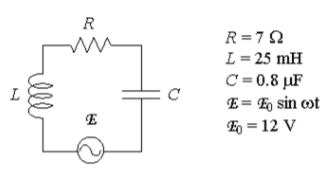# Problem: A series RLC circuit with L = 25 mH, C = 0.8 μF and R = 7Ω is driven by a generator with a maximum emf of 12V and a variable angular frequency ω. At ω = 8000 rad/sec the impedance Z of the circuit is:a) Z = 7 Ωb) Z = 12.3 Ωc) Z = 27.1 Ωd) Z = 44.3 Ωe) Z = 172 Ω

###### Problem Details

A series RLC circuit with L = 25 mH, C = 0.8 μF and R = 7Ω is driven by a generator with a maximum emf of 12V and a variable angular frequency ω. At ω = 8000 rad/sec the impedance Z of the circuit is:

a) Z = 7 Ω

b) Z = 12.3 Ω

c) Z = 27.1 Ω

d) Z = 44.3 Ω

e) Z = 172 Ω